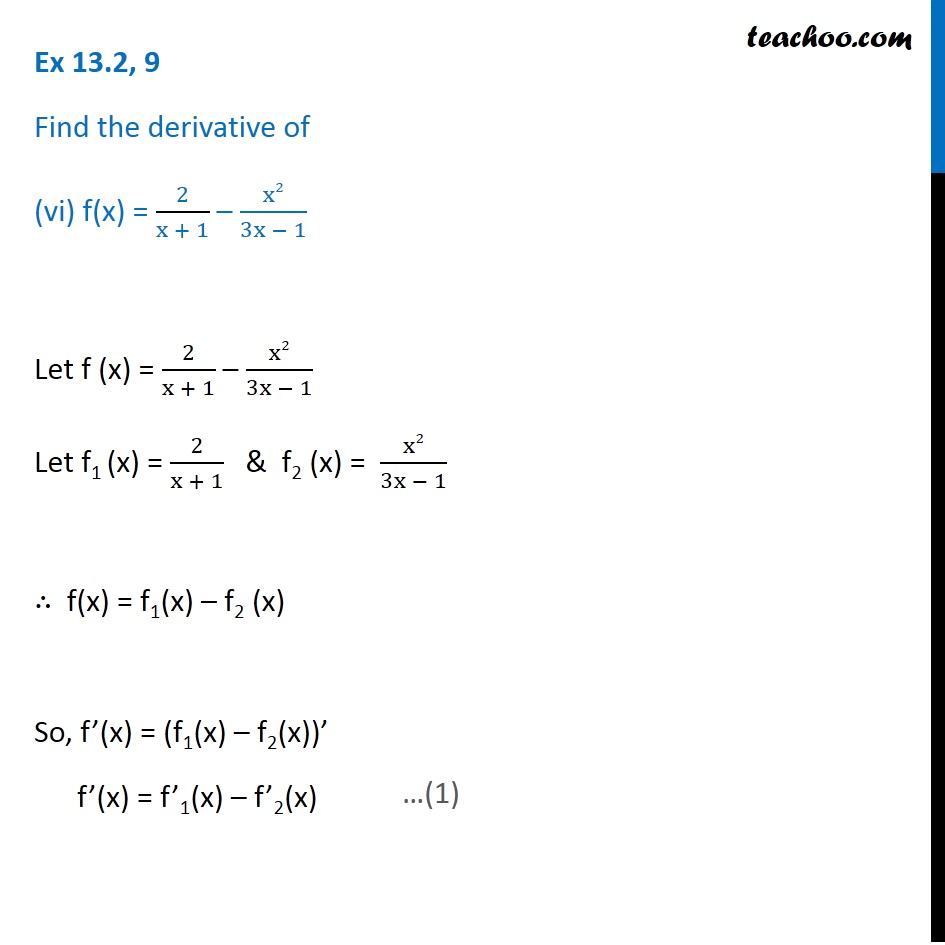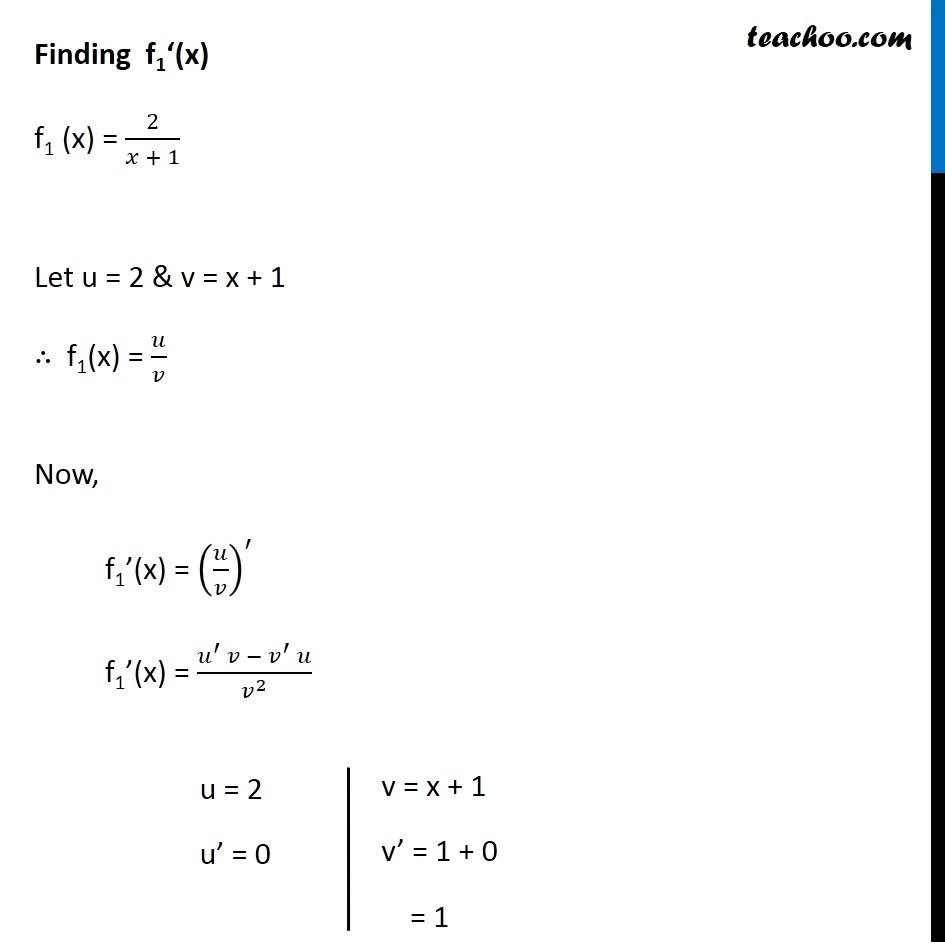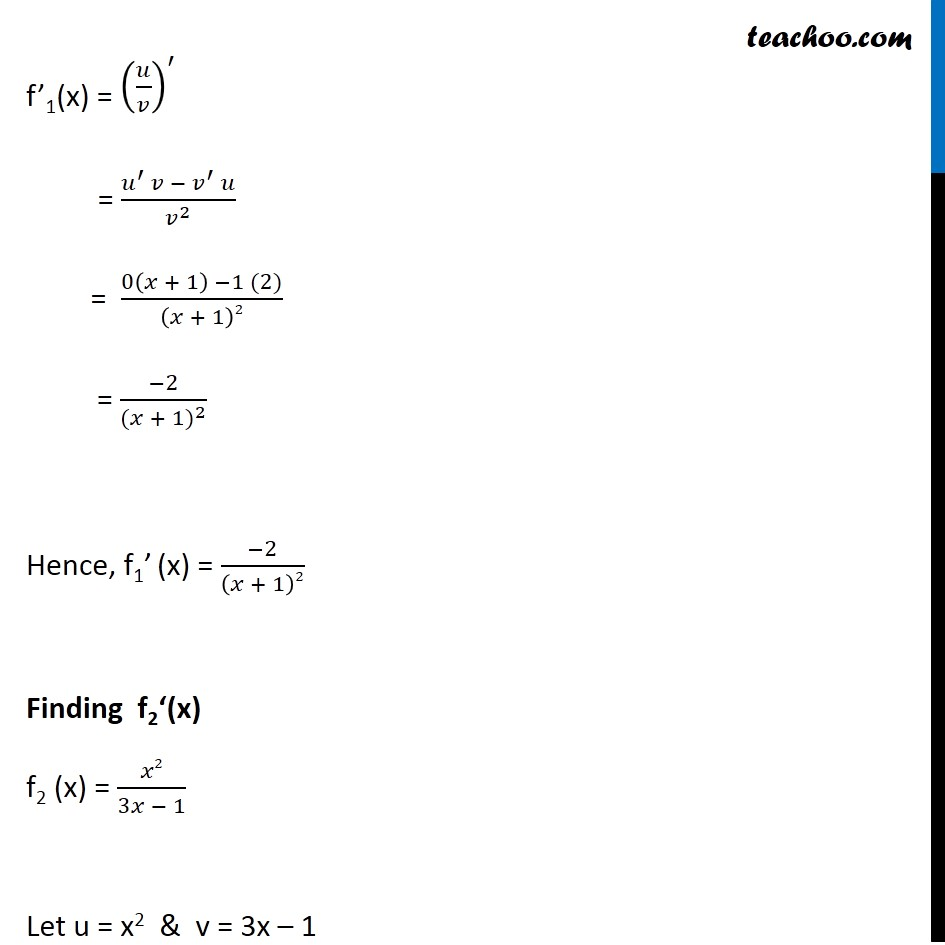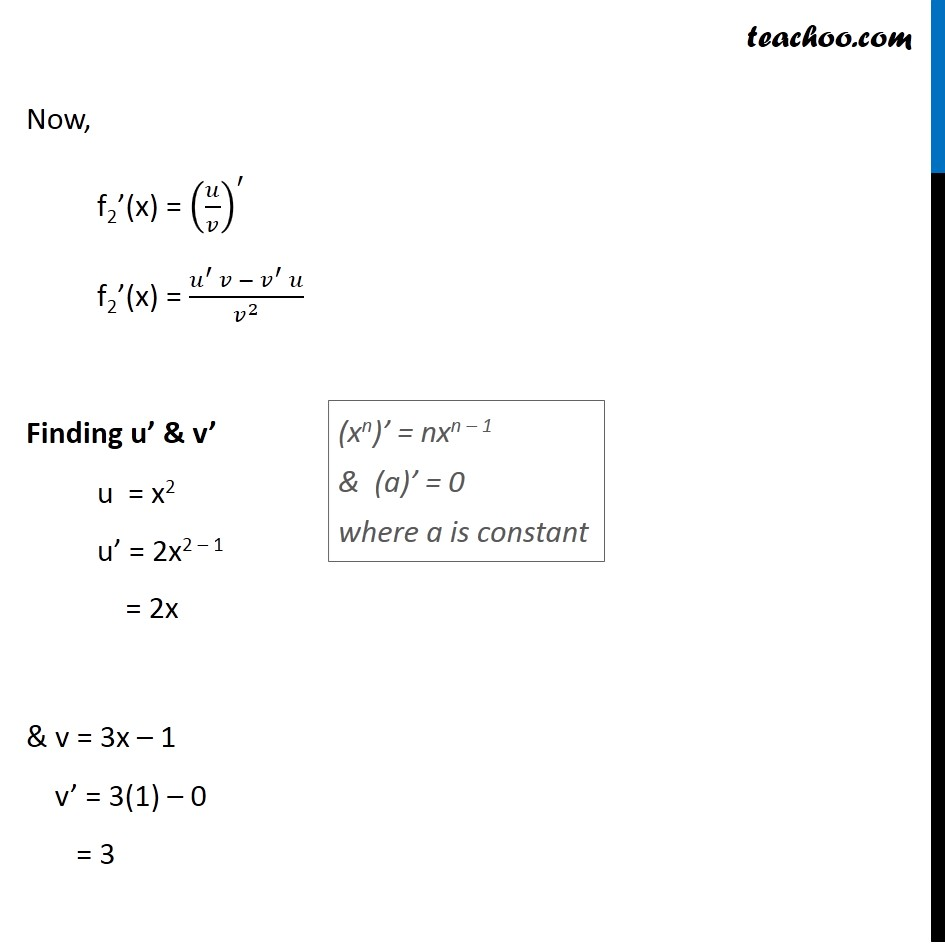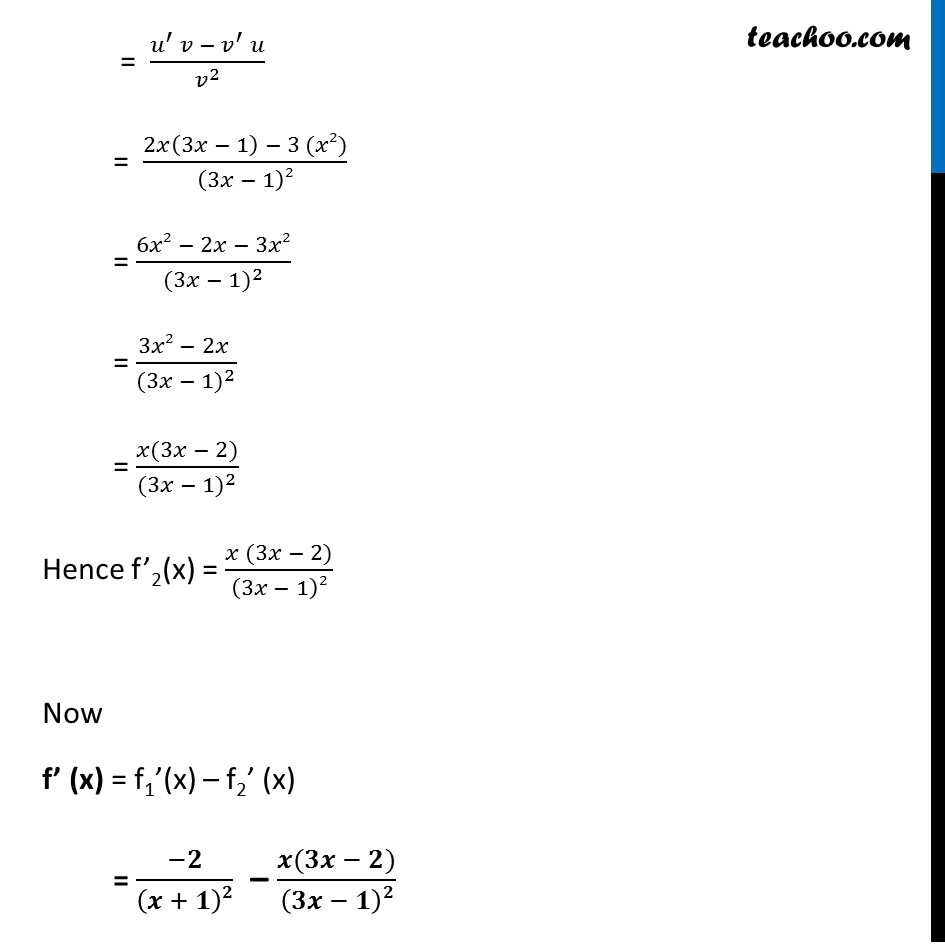1. Chapter 13 Class 11 Limits and Derivatives (Term 1 and Term 2)
2. Serial order wise
3. Ex 13.2 (Term 2)

Transcript

Ex 13.2, 9 Find the derivative of (vi) f(x) = 2/(x + 1) – x2/(3x − 1) Let f (x) = 2/(x + 1) – x2/(3x − 1) Let f1 (x) = 2/(x + 1) & f2 (x) = x2/(3x − 1) ∴ f(x) = f1(x) – f2 (x) So, f’(x) = (f1(x) – f2(x))’ f’(x) = f’1(x) – f’2(x) Finding f1‘(x) f1 (x) = 2/(𝑥 + 1) Let u = 2 & v = x + 1 ∴ f1(x) = 𝑢/𝑣 Now, f1’(x) = (𝑢/𝑣)^′ f1’(x) = (𝑢^′ 𝑣 −〖 𝑣〗^′ 𝑢)/𝑣^2 u = 2 u’ = 0 v = x + 1 v’ = 1 + 0 = 1 f’1(x) = (𝑢/𝑣)^′ = (𝑢^′ 𝑣 −〖 𝑣〗^′ 𝑢)/𝑣^2 = (0(𝑥 + 1) −1 (2))/(𝑥 + 1)2 = (−2)/〖(𝑥 + 1)〗^2 Hence, f1’ (x) = (−2)/(𝑥 + 1)2 Finding f2‘(x) f2 (x) = 𝑥2/(3𝑥 − 1) Let u = x2 & v = 3x – 1 Now, f2’(x) = (𝑢/𝑣)^′ f2’(x) = (𝑢^′ 𝑣 −〖 𝑣〗^′ 𝑢)/𝑣^2 Finding u’ & v’ u = x2 u’ = 2x2 – 1 = 2x & v = 3x – 1 v’ = 3(1) – 0 = 3 f’2(x) = (𝑢/𝑣)^′ (xn)’ = nxn – 1 & (a)’ = 0 where a is constant = (𝑢^′ 𝑣 −〖 𝑣〗^′ 𝑢)/𝑣^2 = (2𝑥(3𝑥 − 1) − 3 (𝑥2))/(3𝑥 − 1)2 = (6𝑥2 − 2𝑥 − 3𝑥2)/〖(3𝑥 − 1)〗^2 = (3𝑥2 − 2𝑥 )/〖(3𝑥 − 1)〗^2 = (𝑥(3𝑥 − 2))/〖(3𝑥 − 1)〗^2 Hence f’2(x) = (𝑥 (3𝑥 − 2))/(3𝑥 − 1)2 Now f’ (x) = f1’(x) – f2’ (x) = (−𝟐)/(𝒙 + 𝟏)𝟐 – (𝒙(𝟑𝒙 − 𝟐))/(𝟑𝒙 − 𝟏)𝟐